## A mass on a spring vibrates in simple harmonic motion at a frequency of 4.0 Hz and an amplitude of 8.0 cm. If the mass of the object is 0.20

Question

A mass on a spring vibrates in simple harmonic motion at a frequency of 4.0 Hz and an amplitude of 8.0 cm. If the mass of the object is 0.20 kg, what is the spring constant

in progress 0
4 weeks 2021-08-17T12:53:14+00:00 1 Answers 0 views 0

The spring has a constant of 126.334 newtons per meter.

Explanation:

Given that mass-spring system experiment a simple harmonic motion, the angular frequency of the system as a function of frequency is: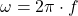Where: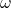– Angular frequency, measured in radians per second.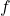– Frequency, measured in hertz.

Given that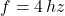, the angular frequency of the system is: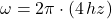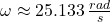Now, the angular frequency can be obtained in terms of spring constant and mass. That is: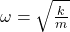Where: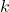– Spring constant, measured in newtons per meter.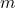– Mass, measured in kilograms.

The spring constant is now cleared: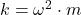If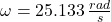and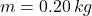, the spring constant is: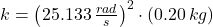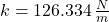The spring has a constant of 126.334 newtons per meter.